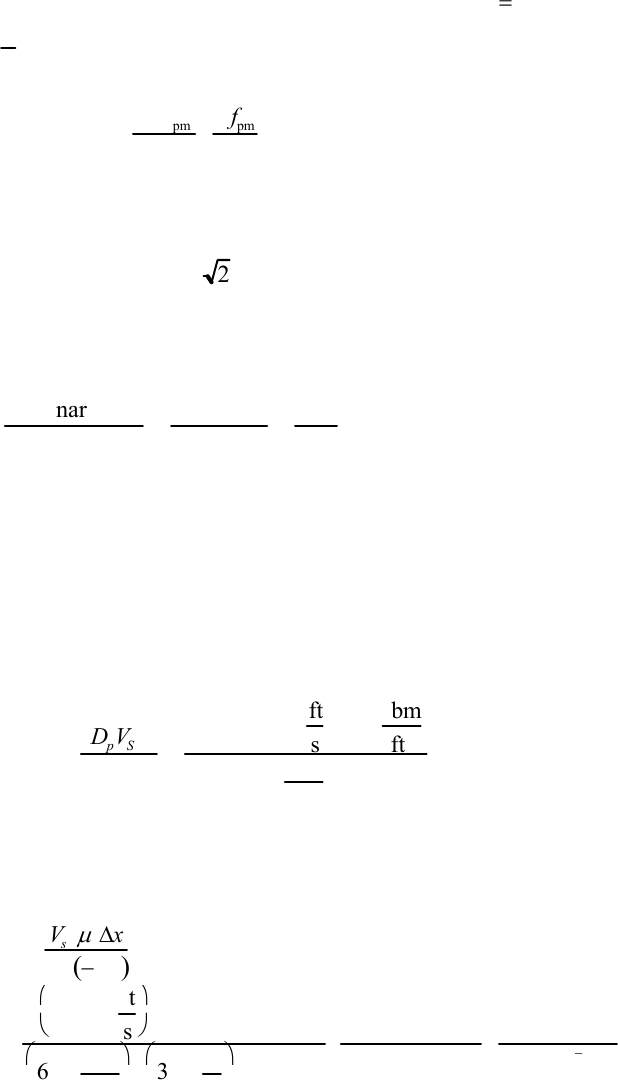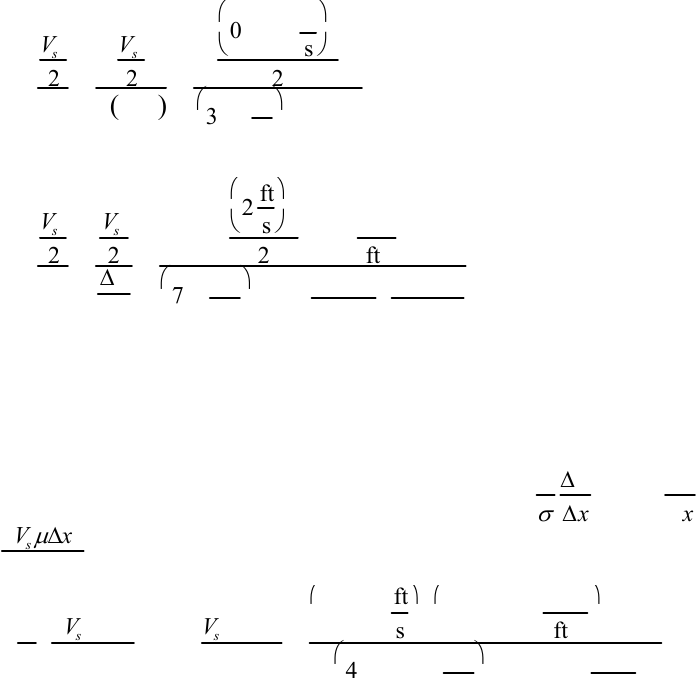Study Guides (400,000)
US (230,000)
LSU (10,000)
CHE (50)
All (10)
Final

# Ch 11Exam

Department
Chemical Engineering
Course Code
CHE 3101
Professor
All
Study Guide
Final

This preview shows page 1. to view the full 5 pages of the document.Solutions, Chapter 11
______________________________________________________________________
11.1
f
pipe Rpipe =16
See the discussion below Eq. 11.10, which shows that
f
pipe
=
3
f
pm and that
Rpipe =2
3
Rpm .
Substituting these, we find
fpmRpm =16 3Rpm
2Rpipe
3
f
pm
fpipe
=72
______________________________________________________________________
11.2 From Eq. 11.14 we see that in laminar flow in porous media
FVs⋅Δ
x
If both
Vs and Δx are multiplied by 2 then their product must be multiplied by 2.
______________________________________________________________________
11.3 The Ergun Eq. (Eq. 11.17) shows f as the sum of that for laminar and for turbulent
flow. The ratio of the laminar term to the turbulent one is
lamina
r
term
turbulent term =150
Rpm /1.75=85.7
Rpm
For = 0.1 this ratio is 857, and the turbulent term is 0.3 % 0 of the sum. Increasing
the by steps of 10 makes the ratio become 85.7, 8.57, 0.857, 0.0857, 0.00857. Thus
at = 10,000, the laminar term is 0.14% 0 of the sum.
Rpm
Rpm
Rpm
I know of no theoretical justification for adding the two terms to get a predictive
curve in the transition region. However it represents experimental data satisfactorily and
is widely used.
______________________________________________________________________
11.4
RP.M. =DpVS
ρ
μ
(1
ε
)=
0.0025 ft 2f
t
s62.3 lb
m
ft3
6.73104lbm
ft s 10.033
()
=690
This is at the high end of the transition region, practically all turbulent.
______________________________________________________________________
11.5* k=Vs
μ
Δ
ρ
gΔz()
=
0.0124 f
t
s
1.002cp()1ft()
62.3 lbm
ft2
32.2 ft
s2
1.25 ft
()
6.72 104lbm
ft s cp darcy
1.06 1011 ft =315 darcies
Solutions, Fluid Mechanics for Chemical Engineers, Third Edition, Chapter 11, page 1

Unlock to view full version

Only page 1 are available for preview. Some parts have been intentionally blurred.This is a large permeability. Most aquifers and petroleum reservoir rocks have
permeabilities of one darcy or less.
______________________________________________________________________
11.6 Here one can argue whether to apply B.E. inside the bed, and use the interstitial
velocity or apply it to the bed outlet, and use the superficial velocity. Doing the latter we
find for Ex. 11.1
Vs
2
2
F=
Vs
2
2
g−Δz()
=
0.0124 ft
s
2
2
32.2 ft
s2
1.25 ft
()
=1.9 106
and for Ex. 11.2
Vs
2
2
F=
Vs
2
2
ΔP
ρ
=
2ft
s
2
262.3 lbm
ft3
701 lbf
in2
32.2 lbf ft
lbm s2144 in2
ft2
=1.9106
If one used interstitial, one would find the above answers multiplied by (1/0.33)2 = 9.1.
The small values here should convince the students that in normal porous media flows we
can ignore the kinetic energy terms.
______________________________________________________________________
11.7* To use Fig. 11.5 we need the capillary number. Ca =
k
σ
Δ
P
Δ
x
Here Δ
P
Δ
=g
ρ
and
k=
V
s
μ
Δ
x
ρ
g(−Δz) combining these we have
Ca =1
σ
Vs
μ
Δx
ρ
g(−Δz)
ρ
g=Vs
μ
Δx
σ
Δz=
0.0124 f
t
s
2.09 105lbf s
ft 2
1ft
()
4.15 104lbf
in
1.25 ft
()
12 in
1ft
=4.17105
Then from Fig. 11.5 we can read the residual saturation as about 0.16
______________________________________________________________________
11.8 If the bed were filled completely with water, the value on the abscissa would be 12
inches/ft. Thus in the "flooded" condition at E, the packing's void spaces are only 50 to
60% full of water.
______________________________________________________________________
11.9* (a) At the low end of the curve,
ρ
Vs = 5 lbm/ft2hr and
Solutions, Fluid Mechanics for Chemical Engineers, Third Edition, Chapter 11, page 2